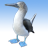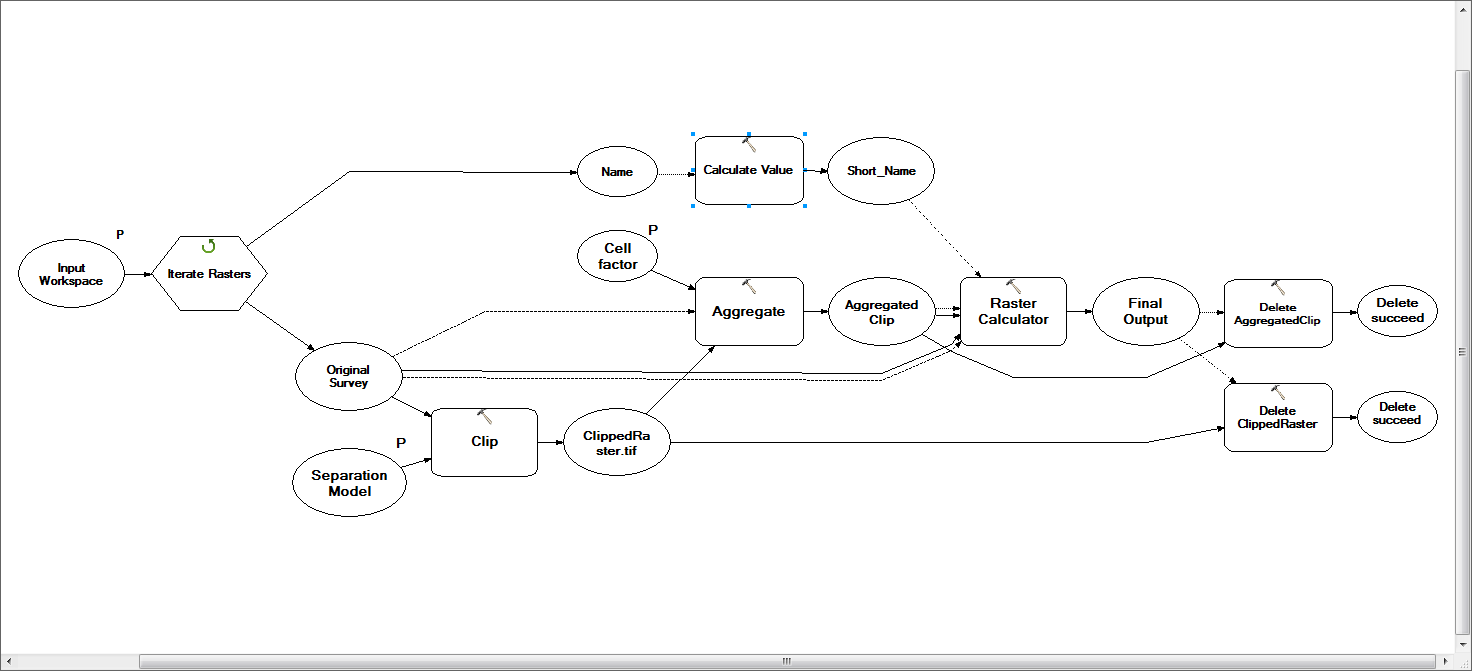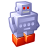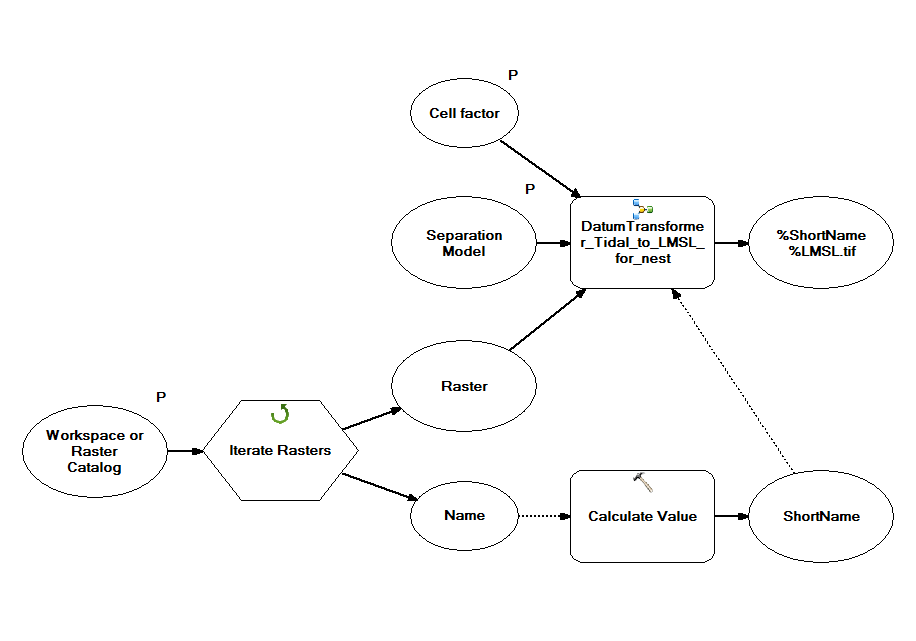# Model Builder angst - can't get Calculate Value tool to iterate along with rest of the model

3122
4
08-06-2015 12:09 PMNew Contributor II

I am trying to create a model that iterates through a directory of rasters and performs a series of raster functions and whose final output is a raster. This seems simple enough except for the fact that I want to use a truncated version of the input raster name as an inline variable to name the output raster.

As you can see from my model, my approach to this is to use the Calculate Value tool on the Name variable that is an automatic output of the Iterate Rasters iterator. the expression I'm using in Calculate Value is "%Name%"[0:7]. The result of running this model is that the first two output rasters in the iteration process seem to be processed correctly and written with the correct output names. After the first two, however, the first output raster is constantly overwritten by the rasters passing through the model in subsequent iterations. I'm wondering if this is because the Calculate Value tool is not actually iterating because, while the Name value is a precondition of the Calculate Value function, it is not actually and input and therefore does not trigger Calculate Value to iterate with the rest of the model. Any help with how to force it to do this or other means to the same end would be greatly appreciated!4 RepliesRegular Contributor II

Could you upload your model and a small sample of data so that I can take a look at this?byMVP Notable Contributor

Just an idea make the output of the Aggregate tool the precondition to the Calculate Value tool?byMVP Esteemed Contributor

The precondition should force execution on every iteration, just like an input variable does.

Duncan would be VERY correct if the output of the Calculate Value is being used to name the output of the Aggregate tool.New Contributor II

I actually ended up solving this issue by creating a nested model with the iterator on the outside, like so:I will run the previous model with the precondition as suggested by Duncan and Curtis to see if that also works. Thanks!